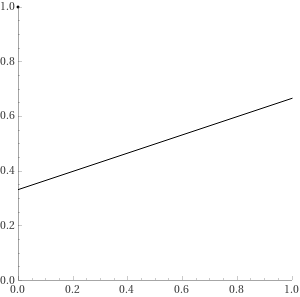Search IntMath
Close

# Distance Between Two Parallel Lines in Geometry## What are Parallel Lines?

Parallel lines are lines that lie in the same plane and never intersect, no matter how far they are extended. In geometry, parallel lines are the same distance apart and never touch each other. Parallel lines have slopes that are equal and are always going in the same direction.

## Coordinates and Distance

In order to calculate the distance between two parallel lines, we must first identify the coordinates of each line. The coordinates of a line can be determined by its slope and a point on the line. The equation of two parallel lines is y = mx + c, where m is the slope and c is the y-intercept. The distance between two parallel lines is the vertical distance between the two lines.

## Perpendicular Lines

Perpendicular lines are lines that intersect at a 90-degree angle. The distance between two perpendicular lines can be calculated by using the equation d = 1/m, where m is the slope of the line. For example, if the slope of one line is 3, then the distance between the two lines is 1/3.

## Calculating Distance Between Two Parallel Lines

The distance between two parallel lines can be calculated by finding the difference between the y-intercepts of the two lines. The equation for finding the distance between two parallel lines is d = |c2 - c1|, where c2 is the y-intercept of line 2 and c1 is the y-intercept of line 1. For example, if the y-intercepts of two lines are -2 and 4, then the distance between them is 6.

## Practice Problems

1. Find the distance between the lines y = 3x � 4 and y = 3x + 2.

Answer: The distance between the lines is 6.

2. Find the distance between the lines y = -2x + 6 and y = -2x + 10.

Answer: The distance between the lines is 4.

3. Find the distance between the lines y = 4x + 3 and y = 4x � 1.

Answer: The distance between the lines is 4.

4. Find the distance between the lines y = -5x + 8 and y = -5x � 4.

Answer: The distance between the lines is 12.

5. Find the distance between the lines y = -3x + 1 and y = -3x + 7.

Answer: The distance between the lines is 6.

6. Find the distance between the lines y = 2x � 5 and y = 2x + 3.

Answer: The distance between the lines is 8.

7. Find the distance between the lines y = 6x + 2 and y = 6x � 8.

Answer: The distance between the lines is 10.

## Summary

In this lesson, we discussed how to calculate the distance between two parallel lines in geometry. We learned that the distance between two parallel lines is the vertical distance between the two lines, which can be calculated by finding the difference between the y-intercepts of the two lines. We also discussed how to calculate the distance between two perpendicular lines. Finally, we practiced calculating the distance between two parallel lines with a few practice problems.

## FAQ

### What is the distance between two parallel lines?

The distance between two parallel lines is always the same and is equal to the length of the perpendicular line connecting them.

### How is the distance determined?

The distance between two parallel lines can be determined by measuring the length of the perpendicular line connecting them.

## Problem SolverThis tool combines the power of mathematical computation engine that excels at solving mathematical formulas with the power of GPT large language models to parse and generate natural language. This creates math problem solver thats more accurate than ChatGPT, more flexible than a calculator, and faster answers than a human tutor. Learn More.